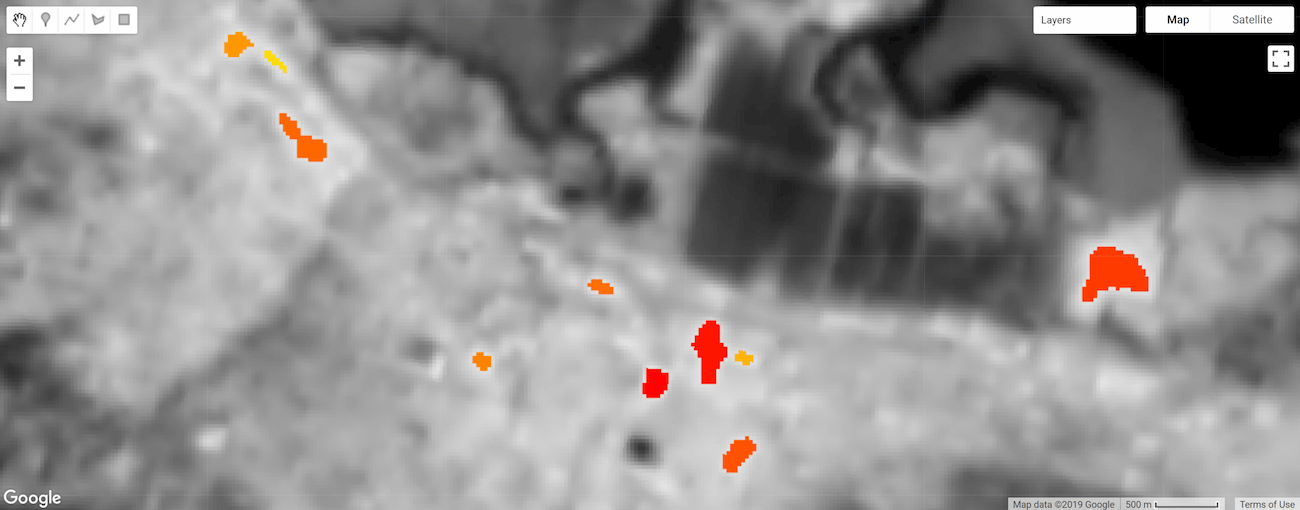# Object-based methods

Image objects are sets of connected pixels having the same integer value. Categorical, binned, and boolean image data are suitable for object analysis.

Earth Engine offers methods for labeling each object with a unique ID, counting the number of pixels composing objects, and computing statistics for values of pixels that intersect objects.

## Example setup

The following sections provide examples of object-based methods applied to Landsat 8 surface temperature with each section building on the former. Run the next snippet to generate the base image: thermal hotspots (> 303 degrees Kelvin) for a small region of San Francisco (Figure 1).

```// Make an area of interest geometry centered on San Francisco.
var point = ee.Geometry.Point(-122.1899, 37.5010);
var aoi = point.buffer(10000);

// Import a Landsat 8 image, subset the thermal band, and clip to the
// area of interest.
var kelvin = ee.Image('LANDSAT/LC08/C01/T1_TOA/LC08_044034_20140318')
.select(['B10'], ['kelvin'])
.clip(aoi);

// Display the thermal band.
Map.centerObject(point, 13);
Map.addLayer(kelvin, {min: 288, max: 305}, 'Kelvin');

// Threshold the thermal band to set hot pixels as value 1 and not as 0.
var hotspots = kelvin.gt(303)
// Mask the cold pixels (value 0).
.rename('hotspots');

// Display the thermal hotspots on the Map.
```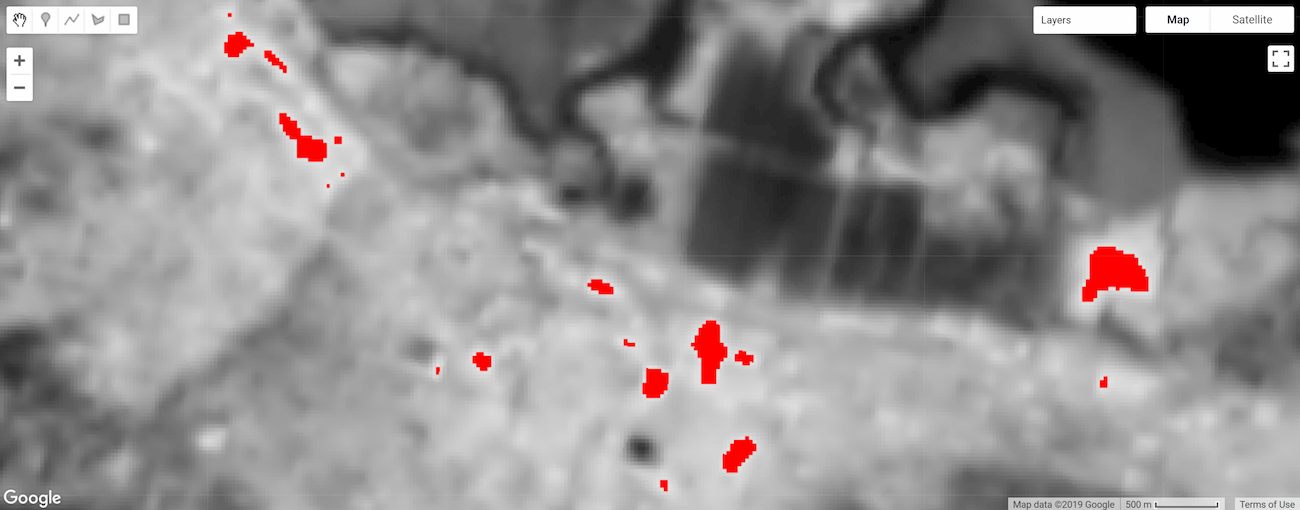Figure 1. Temperature for a region of San Francisco. Pixels with temperature greater than 303 degrees Kelvin are distinguished by the color red (thermal hotspots).

## Label objects

Labeling objects is often the first step in object analysis. Here, the `connectedComponents()` function is used to identify image objects and assign a unique ID to each; all pixels belonging to an object are assigned the same integer ID value. The result is a copy of the input image with an additional "labels" band associating pixels with an object ID value based on connectivity of pixels in the first band of the image (Figure 2).

```// Uniquely label the hotspot image objects.
var objectId = hotspots.connectedComponents({
connectedness: ee.Kernel.plus(1),
maxSize: 128
});

// Display the uniquely ID'ed objects to the Map.
```

Note that the maximum patch size is set to 128 pixels; objects composed of more pixels are masked. The connectivity is specified by an `ee.Kernel.plus(1)` kernel, which defines four-neighbor connectivity; use `ee.Kernel.square(1)` for eight-neighbor.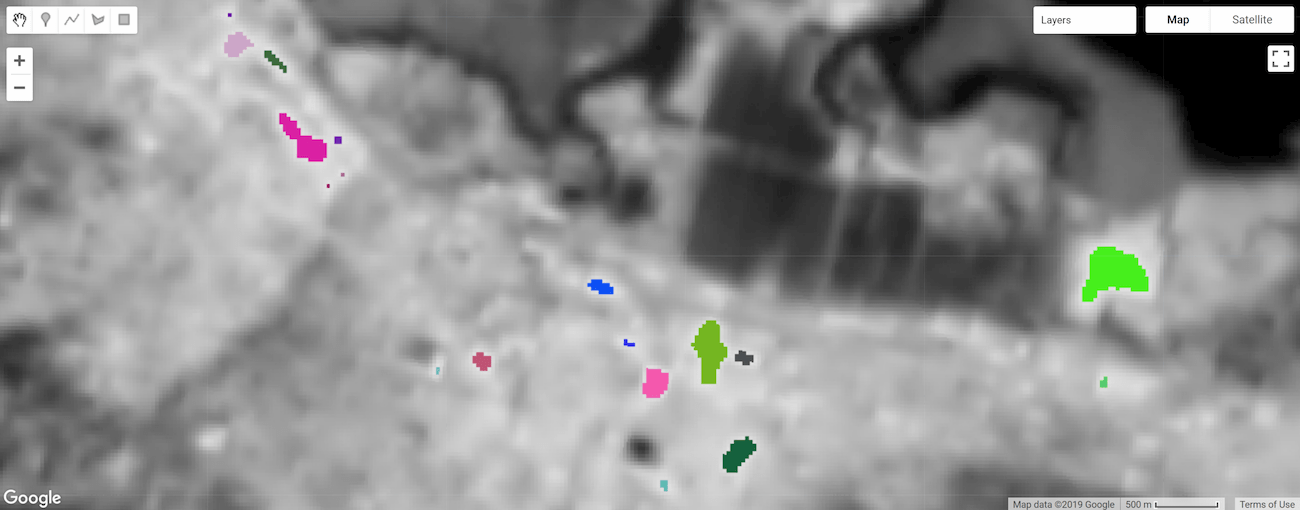Figure 2. Thermal hotspot objects labeled and styled by a unique ID.

## Object size

### Number of pixels

Calculate the number of pixels composing objects using the `connectedPixelCount()` image method. Knowing the number of pixels in an object can be helpful for masking objects by size and calculating object area. The following snippet applies `connectedPixelCount()` to the "labels" band of the `objectId` image defined in the previous section.

```// Compute the number of pixels in each object defined by the "labels" band.
var objectSize = objectId.select('labels')
.connectedPixelCount({
maxSize: 128, eightConnected: false
});

// Display object pixel count to the Map.
```

`connectedPixelCount()` returns a copy of the input image where each pixel of each band contains the number of connected neighbors according to either the four- or eight-neighbor connectivity rule determined by a boolean argument passed to the `eightConnected` parameter. Note that connectivity is determined independently for each band of the input image. In this example, a single-band image (`objectId`) representing object ID was provided as input, so a single-band image was returned with a "labels" band (present as such in the input image), but now the values represent the number of pixels composing objects; every pixel of each object will have the same pixel count value (Figure 3).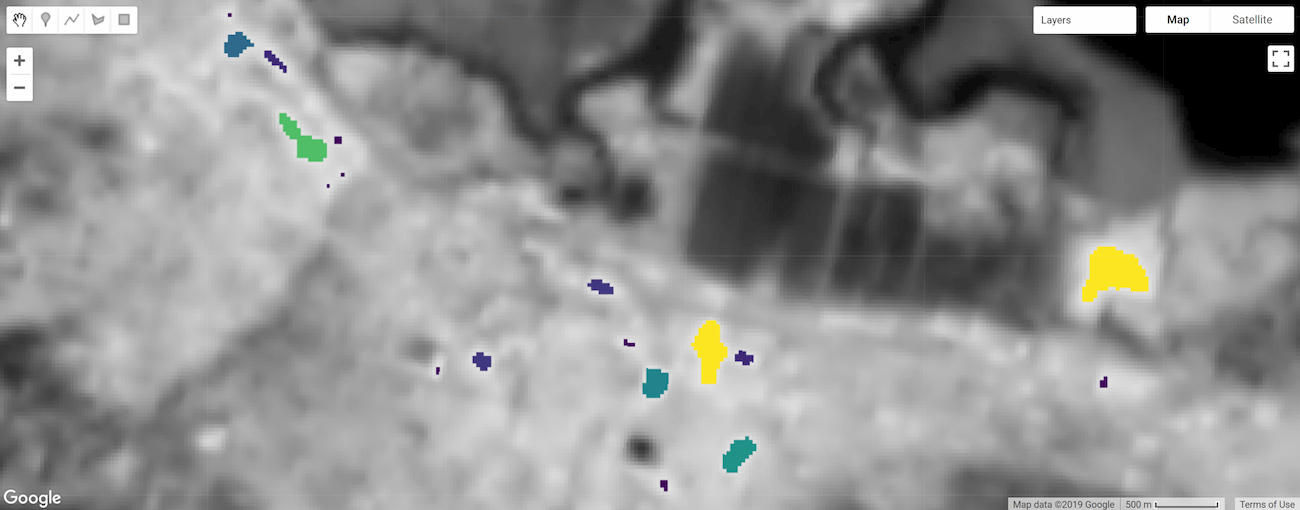Figure 3. Thermal hotspot objects labeled and styled by size.

### Area

Calculate object area by multiplying the area of a single pixel by the number of pixels composing an object (determined by `connectedPixelCount()`). Pixel area is provided by an image generated from `ee.Image.pixelArea()`.

```// Get a pixel area image.
var pixelArea = ee.Image.pixelArea();

// Multiply pixel area by the number of pixels in an object to calculate
// the object area. The result is an image where each pixel
// of an object relates the area of the object in m^2.
var objectArea = objectSize.multiply(pixelArea);

// Display object area to the Map.
```

The result is an image where each pixel of an object relates the area of the object in square meters. In this example, the `objectSize` image contains a single band, if it were multi-band, the multiplication operation would be applied to each band of the image.

## Filter objects by size

Object size can be used as a mask condition to focus your analysis on objects of a certain size (e.g., mask out objects that are too small). Here the `objectArea` image calculated in the previous step is used as a mask to remove objects whose area are less than one hectare.

```// Threshold the `objectArea` image to define a mask that will mask out
// objects below a given size (1 hectare in this case).

// Update the mask of the `objectId` layer defined previously using the
// minimum area mask just defined.
```

The result is a copy of the `objectId` image where objects less than one hectare are masked out (Figure 4).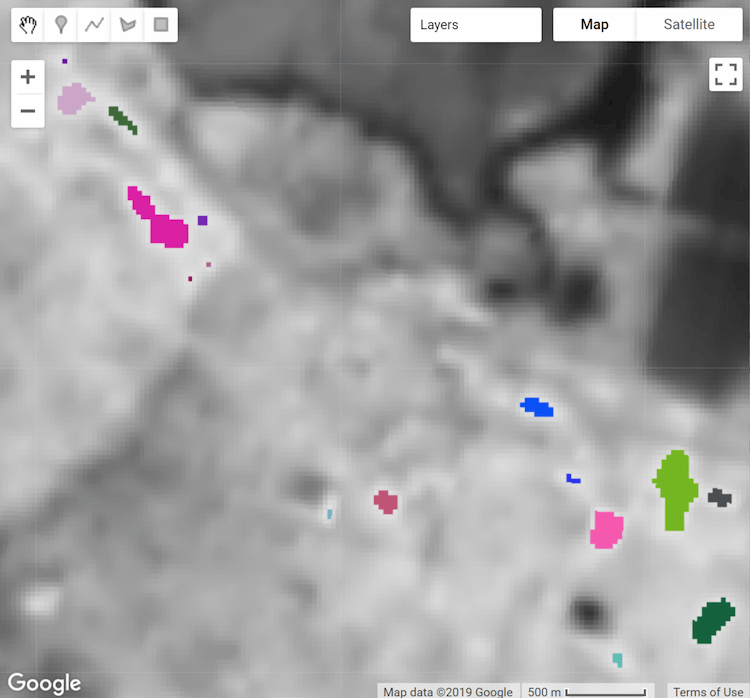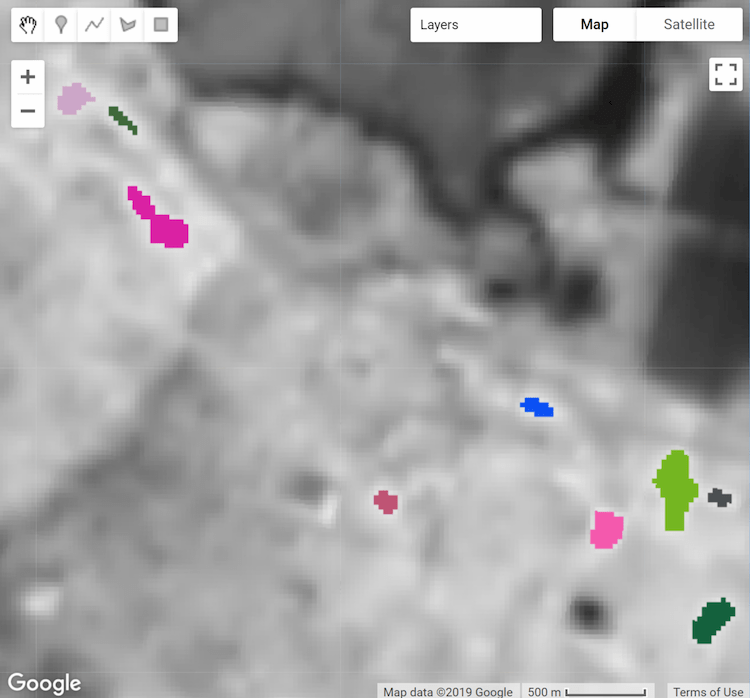Figure 4a. Thermal hotspot objects labeled and styled by unique ID. Figure 4b. Thermal hotspot objects filtered by minimum area (1 hectare).

## Zonal statistics

The `reduceConnectedComponents()` method applies a reducer to the pixels composing unique objects. The following snippet uses it to calculate the mean temperature of hotspot objects. `reduceConnectedComponents()` requires an input image with a band (or bands) to be reduced and a band that defines object labels. Here, the `objectID` "labels" image band is added to the `kelvin` temperature image to construct a suitable input image.

```// Make a suitable image for `reduceConnectedComponents()` by adding a label
// band to the `kelvin` temperature image.

// Calculate the mean temperature per object defined by the previously added
// "labels" band.
var patchTemp = kelvin.reduceConnectedComponents({
reducer: ee.Reducer.mean(),
labelBand: 'labels'
});

// Display object mean temperature to the Map.